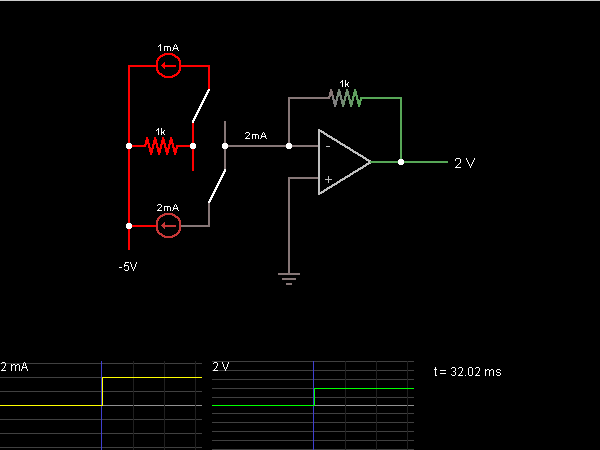# Circuit Simulator - Current-to-Voltage Converter

Current-to-Voltage ConverterCircuit Description:
This circuit converts an input current to a proportional amount of voltage. Use the two switches to select an input current. This current flows across a 1k resistor. The op-amp outputs a voltage equal to the voltage drop across the resistor in order to ensure that the – terminal is at ground, which means that the output voltage is proportional to the resistor voltage (and therefore to the input current).

Discussion:
3 comments Page 1 of 1.

Longin SEBAHIZI said:   9 years ago
Thank you very much for the simulation for the Current to voltage converter.

What type of op-amp used in this converter?

What the output signal of the converter will look like if the input current is AC?
(1)